# Capacitor Theory

By on

Capacitors are widely used in electrical engineering for functions such as energy storage, power factor correction, voltage compensation and many others.  Capacitance is also inherent in any electrical distribution systems and can play a pivotal role in it's operation.

In order to fully understand capacitors and their use, it is essential that electrical practitioners have a good understanding of capacitor theory.

## Capacitance

Symbols Used

C - capacitor, with units of Farad (F)
R - resistor, with units of ohm (Ω)
V - d.c. source voltage in volts (V)
vc - capacitor voltage in volts (V)
I - peak charge or discharge current in amperes (A)
i - instantaneous current in amperes (A)
Q - electric charge (C)
E - electric field strength (V/m)
D - electric flux density (C/m2)
εo - permittivity of free space (f/m) - constant:  8.854 187 817... x 10−12
εr - relative permittivity of dielectric

Capacitors consist of conducting surfaces separated dielectric (insulator).  The effect of this is that when a voltage is applied, charge flows into the capacitor and is stored.  When an external circuit is connected to the capacitor, this stored charge will flow from the capacitor into the circuit.

Capacitance is a measure of amount of charge which can be stored within a capacitor.  The SI unit of capacitance is the farad (F).  The farad is the ratio of electrical charge stored by the capacitor to the voltage applied: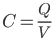The amount of capacitance is defendant on the materials used and geometry of the capacitor.

Formally, capacitance is found by the solution of the Laplace equation ∇2φ = 0, where  φ is a constant potential on conductor surface.   Simpler geometries can also be solved using other methods (the example shows an example for a parallel plate capacitor).

### Example - Parallel Plate Capacitance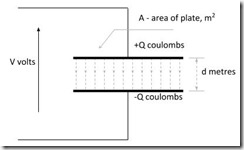Parallel Plate Capacitor
(click for larger image)

Capacitor shown and assume the dielectric is a vacuum.  Electrostatic theory suggests that the ratio of electric flux density to electric field strength is the permittivity of free space: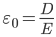The electric flux density and electric field strength are given by:

With capacitance defined as :The above equations can be combined and solved to give the capacitance of a parallel plate capacitor (with a free air dielectric) as:

For more real dielectrics the capacitance will be increased directly in proportion to the the relative permittivity and given by:

## Charging and Discharging of Capacitors

Charging (and discharging) of capacitors follows an exponential law.  Consider the circuit which shows a capacitor connected to a d.c. source via a switch.  The resistor represents the leakage resistance of the capacitor, resistance of external leads and connections and any deliberately introduced resistance.

Capacitor Charging Voltage

When the switch is closed, the initial voltage across the capacitor (C) is zero and the current (i) is given by: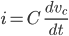- from fundamental capacitor theory

The voltage across the resistor is the current multiplied by its value, giving: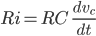From Kirchhoff's voltage law, the d.c. source voltage (V) equals the sum of the capacitor voltage (vc) and voltage across the resistor:

Which when rearranged gives:

By integrating both sides, we get: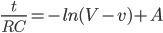By rearranging

The voltage across the capacitor will increase from zero to that of the d.c. source as an exponential function.

### Capacitor Charging  Current

From the above: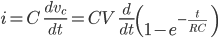Giving: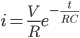Letting the initial current (I), be the d.c source voltage divided by the resistance:

### Time Constant

The product of resistance and capacitance (RC), has the units of seconds and is refereed to as the circuit time constant (denoted by the Greek letter Tau, τ).

Using this, the equations of voltage and charging current across the capacitor are written as: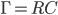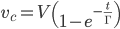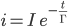Note: increasing the value of resistance R, will increase the time constant resulting in a slower charge (or discharge) of the  capacitor.

### Capacitor Discharging

When discharging the current behaves the same as that for charging, but in the opposite direction.  Voltage across the capacitor will decay exponentially to zero.  Equations for both current and voltage discharge can be determined in a similar way to that shown above and are summarized as: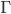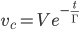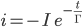## Energy Storage

The greater the capacitance, the more energy it can store.

Current in the capacitor is given by: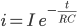Instantaneous power within the capacitor is the product of current and voltage:

During an interval dt, the energy supplied is:

By integrating the instantaneous energy as the capacitor voltage rises, we can find the total energy stored:

It is worth noting that when connecting capacitors in series, the total capacitance reduces but the voltage rating increases.  Connecting in parallel keeps the voltage rating the same, but increases the total capacitance.  Either way the total energy storage of any combination is simply the sum of the storage capacity of each individual capacitor.

### Resistor Losses

In charging an ideal capacitor there are no losses.  However, should a capacitor be charged via a resistor then it should be understood that half of the charging energy will be lost and dissipated as heat across the capacitor.

Consider the above circuit, with a charging current of:The instantaneous power loss across the resistor is:

Consequentially the total power loss is: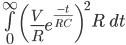Working through the solution gives:

$∫ 0 ∞ V 2 R e −2t RC dt = [ V 2 R ( −RC 2 ) e −2t RC ] 0 ∞ =[ 0 ]−[ − C V 2 2 ]$

$= 1 2 C V 2$ joules

It can be seen that the energy loss is the same as that stored within the capacitor.  On discharging, there will also be half the store energy lost within the resistor.

More interesting Notes:Steven McFadyen

Steven has over twenty five years experience working on some of the largest construction projects. He has a deep technical understanding of electrical engineering and is keen to share this knowledge. About the author

#### View 1 Comments (old system)

1.Notes says:
6/27/2013 2:32 PM

Capacitors have numerous applications in electrical and electronic applications.  This note, examines the use of capacitors to store electrical energy.  The sidebar shows details of a typical commercially available energy storage module. ...

Comments are closed for this post:
• have a question or need help, please use our Questions Section
• spotted an error or have additional info that you think should be in this post, feel free to Contact Us

Restricted Earth Fault Protection

The windings of many medium and small sized transformers are protected by restricted earth fault (REF) systems. The illustration shows the principal of...

Alternating Current Circuits

Alternating current (a.c.) is the backbone of modern electrical power distribution. In this article I’ll be pulling some of the more important concepts...

Generation of a Sine Wave

A fundamental concept behind the operation of alternating current systems is that voltage and current waveforms will be sinusoidal – a Sine Wave. This...

Equipment Verification (to IEC Standards)

One of the requirements to ensuring that everything works is to have equipment selected, manufactured and verified [tested] to IEC standards. Not all equipment...

Wiki Depreciation

We have had the Wiki with us for a long time now, but at last I have decided to say bye bye – more details on why below.

Understanding Motor Duty Rating

One of the comments on my Motor Starting Series was asking for something on duty cycles. Here it is. As a purchaser of a motor, you have responsibility...

Bows and Arrows

It starts with me reading one of the Horrible History books with my son (Groovy Greeks). Arrows were mentioned which lead to the discussion of the bodkin...

Why a Sine Wave?

I received this question by email a few weeks. First thoughts was that it is a product of the mathematics of rotating a straight conductor in a magnetic...

Network Theory – Introduction and Review

In electrical engineering, Network Theory is the study of how to solve circuit problems. By analyzing circuits, the engineer looks to determine the various...

myElectrical does not support or promote the use of copyrighted material without the copyright owner's consent. If you believe that material for which...## Have some knowledge to share

If you have some expert knowledge or experience, why not consider sharing this with our community.

By writing an electrical note, you will be educating our users and at the same time promoting your expertise within the engineering community.

To get started and understand our policy, you can read our How to Write an Electrical Note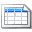Click here to view the notes list in a table formatClick here to view the notes indexed by tag